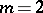# Twins

(diff) ← Older revision | Latest revision (diff) | Newer revision → (diff)
Two primes the difference between which is 2. Generalized twins are pairs of successive primes with difference, whereis a given natural number. Examples of twins are readily found on consulting the table of prime numbers. Such are, e.g., 3 and 5, 5 and 7, 11 and 13, 17 and 19. Generalized twins — for, for example — include 13 and 17, 19 and 23, 43 and 47. It is not yet (1992) known if the set of twins, and even the set of generalized twins for any given, is infinite. This is the twin problem.